# 在 MicroPython 中调用 ujson 内置模块的功能的实例，讲点 MicroPython C 层面的解释执行、异常捕获、内存回收、内置对象等内容。

oh！！！想起来了，刚好可以写一篇关于深度使用 micropython 的文章！会有一些额外的知识讲解哒。

## 起因

#!/usr/bin/python3
import json

# Python 字典类型转换为 JSON 对象
data1 = {
'no' : 1,
'name' : 'Runoob',
'url' : 'http://www.runoob.com'
}

json_str = json.dumps(data1)
print ("Python 原始数据：", repr(data1))
print ("JSON 对象：", json_str)

# 将 JSON 对象转换为 Python 字典
print ("data2['name']: ", data2['name'])
print ("data2['url']: ", data2['url'])


## 理解 MicroPython 的 bytecode (bc) 结构

（以后把图放上来，现在生成的图基本没法看，诺，就跟下面这个一样）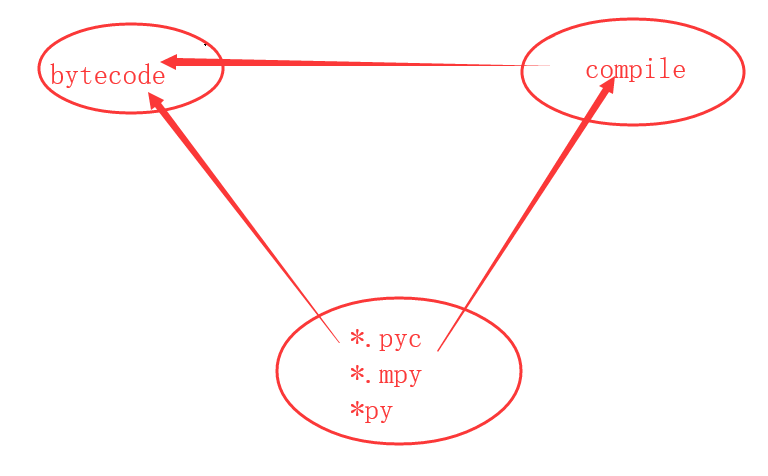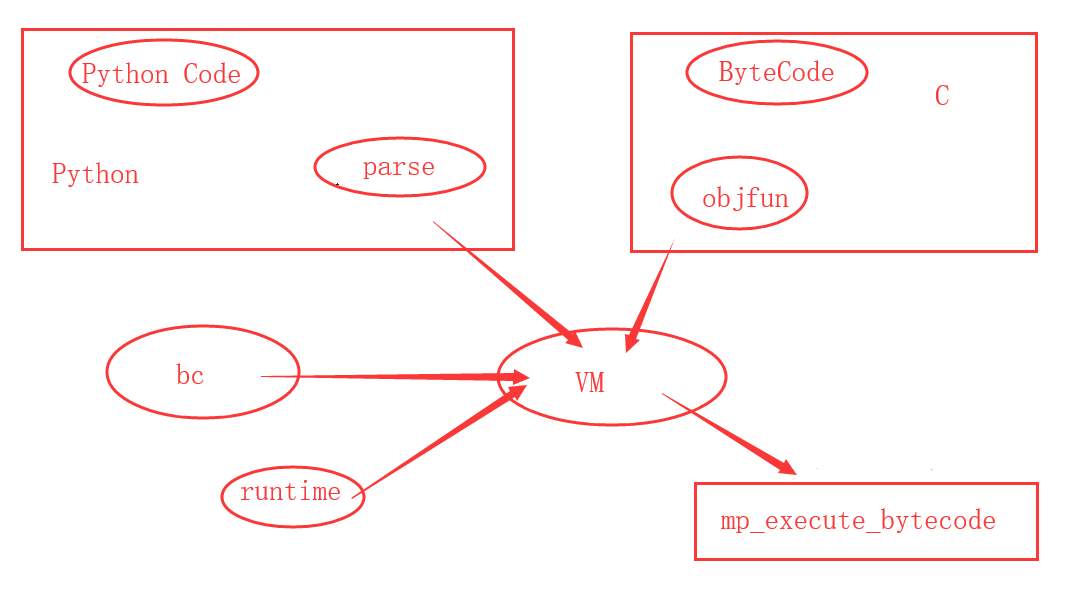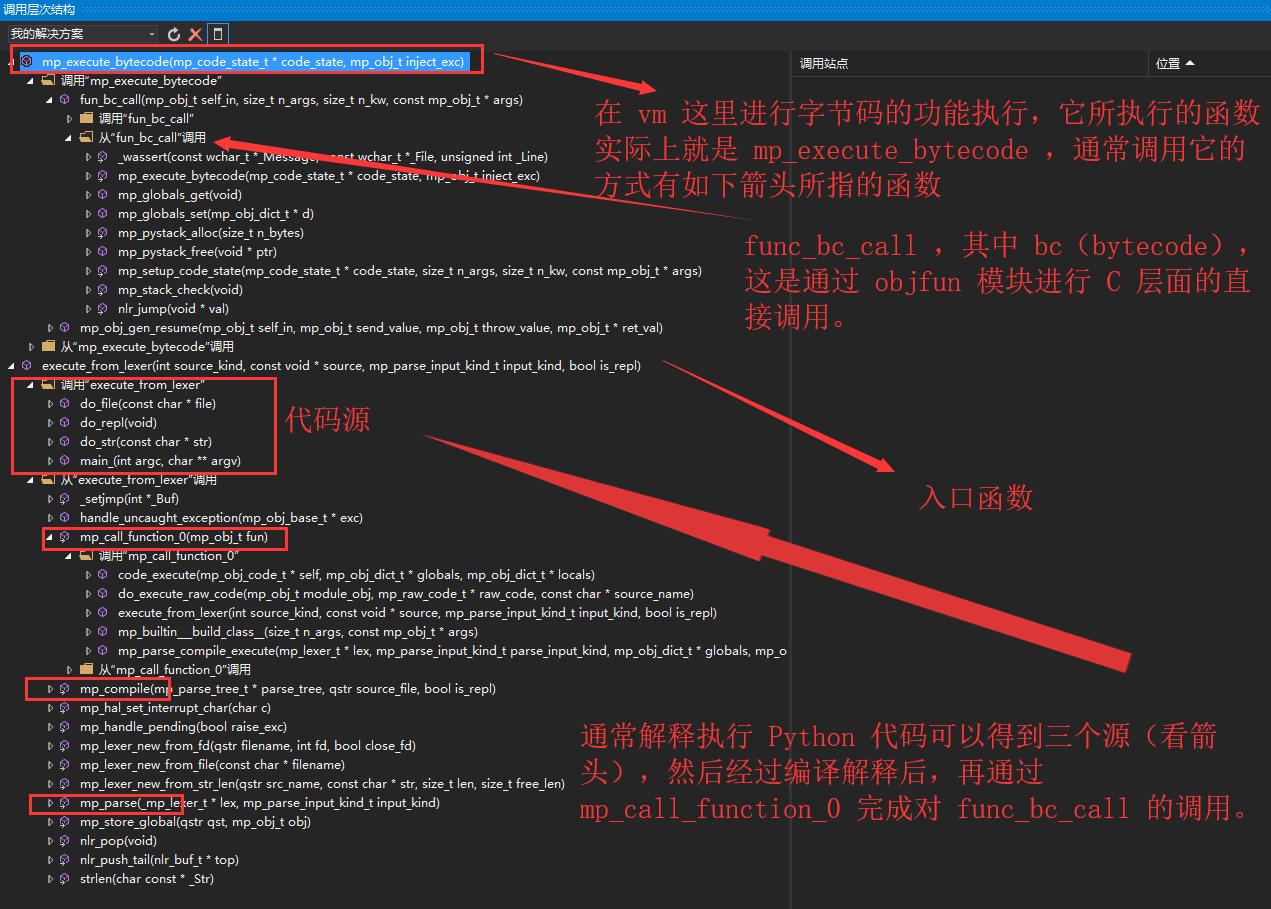## 对 import ujson 调用实例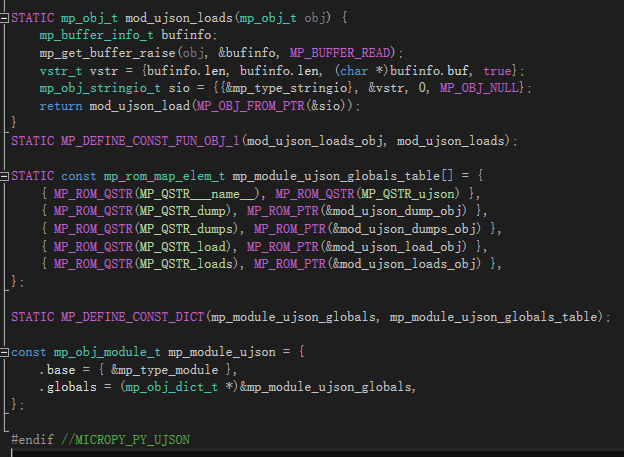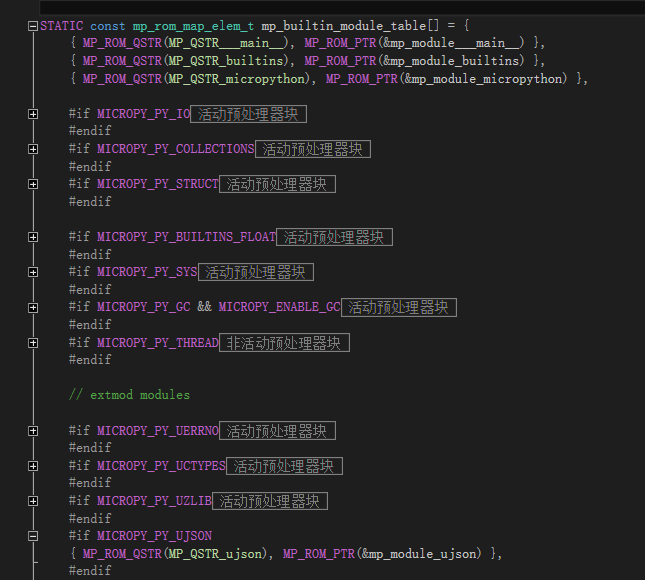import josn
print(result)


• call import josn

mp_obj_t module_obj = (mp_obj_t)mp_module_get(MP_QSTR_ujson);
if (module_obj != MP_OBJ_NULL) {
// import josn
}


• call josn.loads

mp_obj_t dest;
if (dest != MP_OBJ_NULL) {
}


const char json[] = "{\"a\":1,\"b\":2,\"c\":3,\"d\":4,\"e\":\"helloworld\"}";

dest = mp_obj_new_str(json, sizeof(json) - 1);

mp_obj_t result = mp_call_method_n_kw(1, 0, dest);


// args contains: fun  self/NULL  arg(0)  ...  arg(n_args-2)  arg(n_args-1)  kw_key(0)  kw_val(0)  ... kw_key(n_kw-1)  kw_val(n_kw-1)
// if n_args==0 and n_kw==0 then there are only fun and self/NULL
mp_obj_t mp_call_method_n_kw(size_t n_args, size_t n_kw, const mp_obj_t *args) {
DEBUG_OP_printf("call method (fun=%p, self=%p, n_args=" UINT_FMT ", n_kw=" UINT_FMT ", args=%p)\n", args, args, n_args, n_kw, args);
int adjust = (args == MP_OBJ_NULL) ? 0 : 1;
}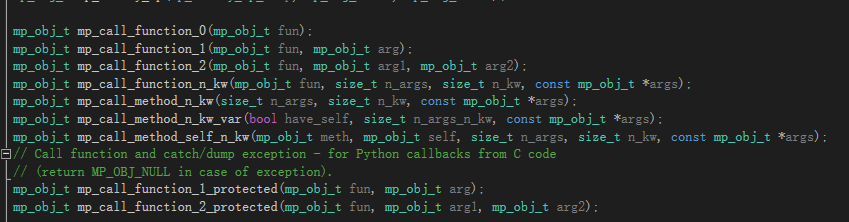• print dict = josn.loads()

## 对 nlr （no local return）的使用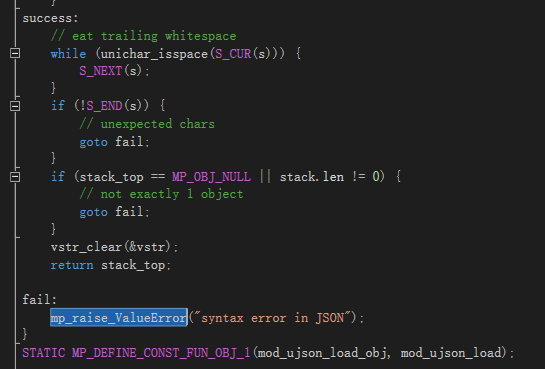import josn
try:
print(result)
except Exception as e:
print(e)


if (nlr_push(&nlr) == 0) {
mp_obj_t result = mp_call_method_n_kw(1, 0, dest);
mp_printf(&mp_plat_print, "print(result)\r\n");
mp_obj_print_helper(&mp_plat_print, result, PRINT_STR);
mp_printf(&mp_plat_print, "\r\n");
nlr_pop();
}
else {
mp_obj_print_exception(&mp_plat_print, (mp_obj_t)nlr.ret_val);
}


## 对 gc 回收内存的机制重建缓存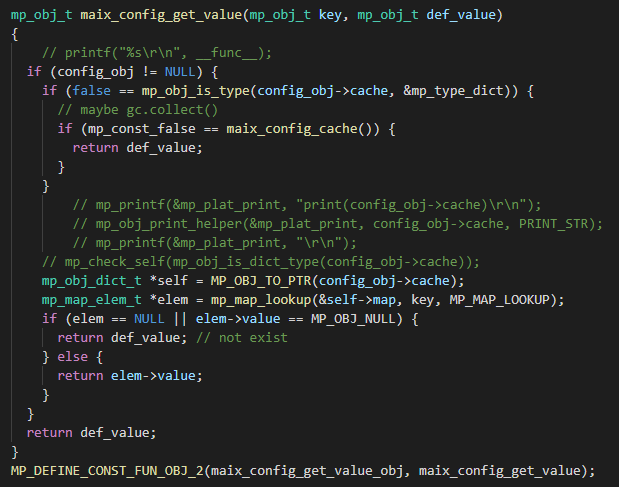if (false == mp_obj_is_type(config_obj->cache, &mp_type_dict)) {
// maybe gc.collect()
if (mp_const_false == maix_config_cache()) {
return def_value;
}
}


## 对 dict 对象（map）的操作

const char goal[] = "your_find_key";
mp_obj_dict_t *self = MP_OBJ_TO_PTR(result);
mp_map_elem_t *elem = mp_map_lookup(&self->map, mp_obj_new_str(goal, sizeof(goal) - 1), MP_MAP_LOOKUP);
mp_obj_t value;
if (elem == NULL || elem->value == MP_OBJ_NULL) {
// not exist
}
else {
value = elem->value;
//mp_check_self(mp_obj_is_str_type(value));
mp_printf(&mp_plat_print, "print(result.get('%s'))\r\n", goal);
mp_obj_print_helper(&mp_plat_print, value, PRINT_STR);
mp_printf(&mp_plat_print, "\r\n");
}



mp_map_elem_t *dict_iter_next(mp_obj_dict_t *dict, size_t *cur) {
size_t max = dict->map.alloc;
mp_map_t *map = &dict->map;

for (size_t i = *cur; i < max; i++) {
if (mp_map_slot_is_filled(map, i)) {
*cur = i + 1;
return &(map->table[i]);
}
}

return NULL;
}

mp_obj_dict_t *self = MP_OBJ_TO_PTR(tmp);
size_t cur = 0;
mp_map_elem_t *next = NULL;
bool first = true;
while ((next = dict_iter_next(self, &cur)) != NULL) {
if (!first) {
mp_print_str(&mp_plat_print, ", ");
}
first = false;
mp_obj_print_helper(&mp_plat_print, next->key, PRINT_STR);
mp_print_str(&mp_plat_print, ": ");
mp_obj_print_helper(&mp_plat_print, next->value, PRINT_STR);
}



## 对接 K210 的特定功能函数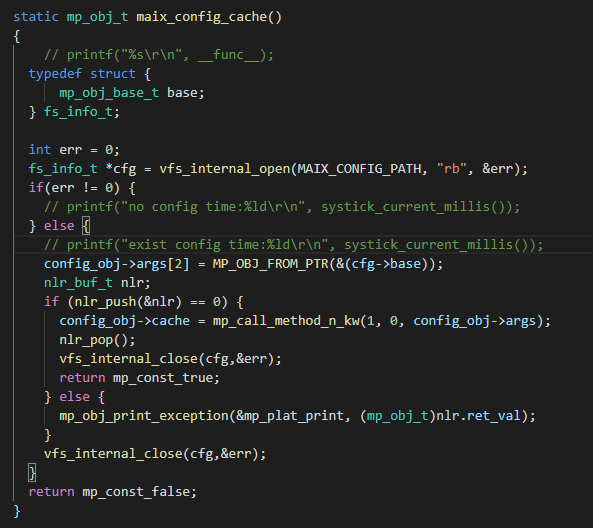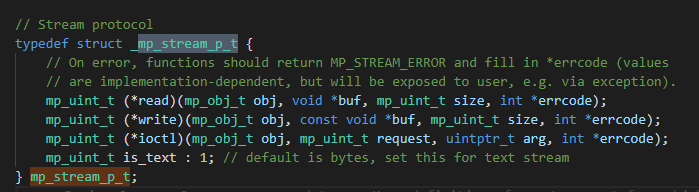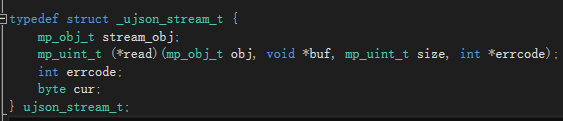# 写入 JSON 数据
with open('data.json', 'w') as f:
json.dump(data, f)

# 读取数据
with open('data.json', 'r') as f: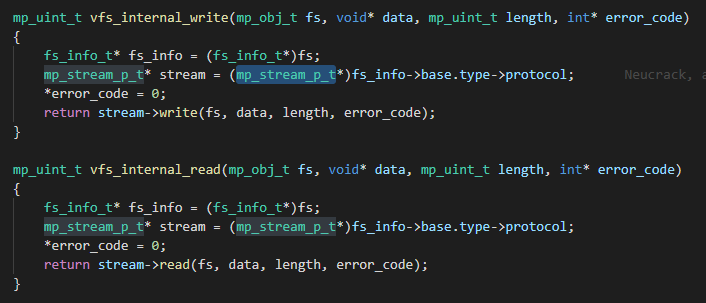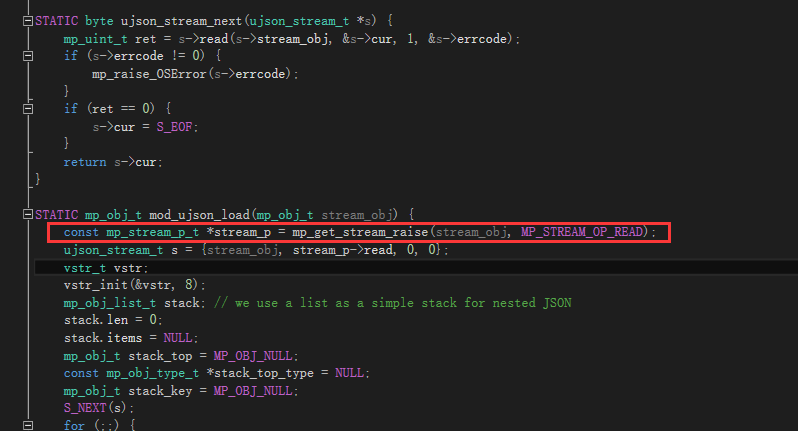## 后记

posted @ 2020-06-09 18:57  Juwan  阅读(592)  评论(0编辑  收藏